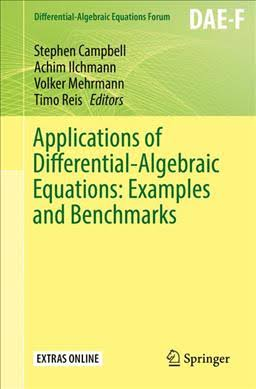# Applications of Differential-Algebraic Equations: Examples and BenchmarksApplications of Differential-Algebraic Equations: Examples and Benchmarks, coedited with Achim Ilchmann, Volker Merhrmann, and Timo Reis. To be published by Springer on March 9, 2019. Available in paper back.

This volume encompasses prototypical, innovative and emerging examples and benchmarks of Differential-Algebraic Equations (DAEs) and their applications, such as electrical networks, chemical reactors, multibody systems, and multiphysics models, to name but a few. Each article begins with an exposition of modelling, explaining whether the model is prototypical and for which applications it is used. This is followed by a mathematical analysis, and if appropriate, a discussion of the numerical aspects including simulation. Additionally, benchmark examples are included throughout the text. Mathematicians, engineers, and other scientists, working in both academia and industry either on differential-algebraic equations and systems or on problems where the tools and insight provided by differential-algebraic equations could be useful, would find this book resourceful.

ISBN to be announced.